MathematicsInterpolation and Multidimensional Arrays

Several interpolation functions operate specifically on multidimensional data.

 Function Description `interp3` Three-dimensional data interpolation. `interpn` Multidimensional data interpolation. `ndgrid` Multidimensional data gridding (`ndfun` directory).

This section discusses:

Interpolation of Three-Dimensional Data

The function `interp3` performs three-dimensional interpolation, finding interpolated values between points of a three-dimensional set of samples `V`. You must specify a set of known data points:

• `X`, `Y`, and `Z` matrices specify the points for which values of `V` are given.
• A matrix `V` contains values corresponding to the points in `X`, `Y`, and `Z`.

The most general form for `interp3` is

• ```VI = interp3(X,Y,Z,V,XI,YI,ZI,`method`)
```

`XI`, `YI`, and `ZI` are the points at which `interp3` interpolates values of `V`. For out-of-range values, `interp3` returns `NaN`.

There are three different interpolation methods for three-dimensional data:

• Nearest neighbor interpolation (`method = 'nearest'`). This method chooses the value of the nearest point.
• Trilinear interpolation (`method = 'linear'`). This method uses piecewise linear interpolation based on the values of the nearest eight points.
• Tricubic interpolation (`method = 'cubic'`). This method uses piecewise cubic interpolation based on the values of the nearest sixty-four points.

All of these methods require that `X`, `Y`, and `Z` be monotonic, that is, either always increasing or always decreasing in a particular direction. In addition, you should prepare these matrices using the `meshgrid` function, or else be sure that the "pattern" of the points emulates the output of `meshgrid`.

Each method automatically maps the input to an equally spaced domain before interpolating. If `x` is already equally spaced, you can speed execution time by prepending an asterisk to the `method` string, for example, `'*cubic'`.

Interpolation of Higher Dimensional Data

The function `interpn` performs multidimensional interpolation, finding interpolated values between points of a multidimensional set of samples `V`. The most general form for `interpn` is

• ```VI = interpn(X1,X2,X3...,V,Y1,Y2,Y3,...,`method`)
```

`1`, `2`, `3`, ...` `are matrices that specify the points for which values of `V` are given. `V` is a matrix that contains the values corresponding to these points. `1`, `2`, `3`, ... are the points for which `interpn` returns interpolated values of `V`. For out-of-range values, `interpn` returns `NaN`.

`Y1`, `Y2`, `Y3`, ... must be either arrays of the same size, or vectors. If they are vectors of different sizes, `interpn` passes them to `ndgrid` and then uses the resulting arrays.

There are three different interpolation methods for multidimensional data:

• Nearest neighbor interpolation (`method = 'nearest'`). This method chooses the value of the nearest point.
• Linear interpolation (`method = 'linear'`). This method uses piecewise linear interpolation based on the values of the nearest two points in each dimension.
• Cubic interpolation (`method = 'cubic'`). This method uses piecewise cubic interpolation based on the values of the nearest four points in each dimension.

All of these methods require that `X1`, `X2,X3 `be monotonic. In addition, you should prepare these matrices using the `ndgrid` function, or else be sure that the "pattern" of the points emulates the output of `ndgrid`.

Each method automatically maps the input to an equally spaced domain before interpolating. If `X` is already equally spaced, you can speed execution time by prepending an asterisk to the `method` string; for example, `'*cubic'`.

Multidimensional Data Gridding

The `ndgrid` function generates arrays of data for multidimensional function evaluation and interpolation. `ndgrid` transforms the domain specified by a series of input vectors into a series of output arrays. The `i`th dimension of these output arrays are copies of the elements of input vector `x``i`.

The syntax for `ndgrid` is

• ```[X1,X2,X3,...] = ndgrid(x1,x2,x3,...)
```

For example, assume that you want to evaluate a function of three variables over a given range. Consider the function

•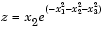for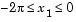,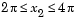, and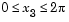. To evaluate and plot this function:

• ```x1 = ```-2:0.2:2;
```x2 = ```-2:0.25:2;
```x3 = -2:0.16:2;
[X1,X2,X3] = ndgrid(x1,x2,x3);
z = X`2.`*exp(-X`1`.^2 -X`2`.^2 -X3.^2);
slice(X`2`,X`1`,X`3`,z,[-1.2 0.8 2],2,[-2 0.2])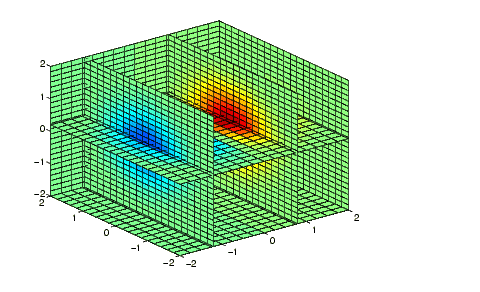```Comparing Interpolation Methods Triangulation and Interpolation of Scattered Data